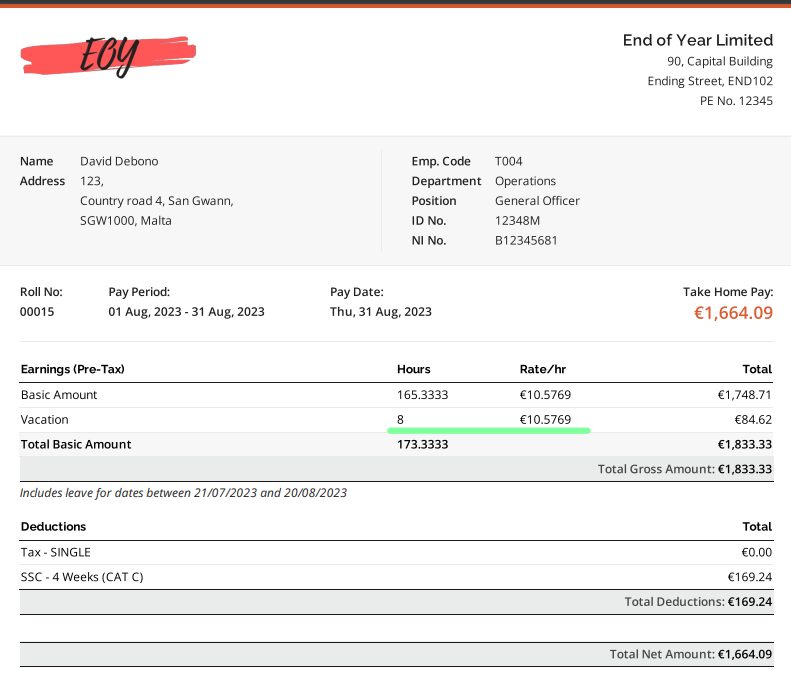# How is the Leave Amount calculated?Talexio Support
• Updated

## Overview

Employees are entitled to a certain amount of leave per year (depending on their job schedule, start dates, accumulated hours, etc.). When leave is consumed, it is added to payroll; but how much is an hour of leave equivalent to in monetary terms?

## Permissions

You need to have the Manage Payroll permission in order to access payroll. To set an employee's remuneration package (and hence, the monetary amount of leave), you will need the Manage employment position history permission.

## How is the leave amount calculated?

Leave amount is based on the hourly rate of the position history that was active at the time when the leave was booked.

So, let's have a look at the below examples

David Debono has an hourly rate of €10.5769.He booked 8 hours of leave on the 18th of August.

August Payroll: Here, as you can see, the 8 hours of leave are being paid at the same rate as the employee's basic hours worked:But what if you earn a yearly amount?

In such a case, the hourly amount is calculated by dividing your yearly salary by the total amount of hours worked in a year.

For example:

A Full Time 40 hour a week employee works 40 hours per week, so 40 hours/week * 52 weeks = 2080 hours/year. Therefore, the hourly amount of an employee earning €20,000 per year is €20,000/2080 = €9.62/hour.

Share:
<% if (previousArticle || nextArticle) { %>
<% if (previousArticle) { %>
<% if (previousTitle) { %>

#### <%= previousTitle %>

<% } %> <%= previousArticle.title %>
<% } %> <% if (nextArticle) { %>
<% if (nextTitle) { %>

#### <%= nextTitle %>

<% } %> <%= nextArticle.title %>
<% } %>
<% } %>
<% if (allItems.length > 1) { %>